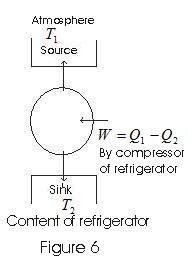# Heat Pump and Principle of Refrigerator

## Heat Pump and Principle of Refrigerator

• Refrigerators works in reverse direction of heat engines.
• In refrigerators working substance extracts heat Q2 from sink at lower temperature T2
• Some external work is performed by the compressor of refrigerator and then heat Q1 is rejected to the
source, to the radiator of the refrigerator.
• A heat pump is the same as a refrigerator. What term we use depends on the purpose of the device. If the purpose is to cool a portion of space, like the inside of a chamber, and higher temperature reservoir is surrounding, we call the device a refrigerator.

if the idea is to pump heat into a portion of space (the room of the building where outside temperature is colder),the device is called the heat pump
•Coefficient of performance :
$\alpha= \frac {\text{Amount of heat absorbed from the cold reservoir}}{\text{work done in running the machinery}}$
$Q_2$ - heat absorbed from cold reservoir.
$Q_1$ - heat rejected to hot reservoir during one complete cycle
$W = Q_1-Q_2$ is the work done in running the machinery
thus,
$\alpha= \frac {Q_2}{W} =\frac {Q_2}{Q_1-Q_2}$
• Like heat engines ,refrigerators can not work without some external work done on the system. Hence coefficient of performance can not be infinite.

## Solved Example

Question 1
If a refrigerator's door is kept open, will the room become cool or hot? Explain.
Solution
If a refrigerator door is kept open the room will become hotter, because amount of heat absorbed from inside the refrigerator and work done on refrigerator by electricity both will be rejected by refrigerator in room.